Pythagorean triples

(note by R. Bigoni)

Pythagorean triples

A Pythagorean triple is a sequence of three natural numbers a, b and c such that a2+b2=c2.

If a and b are relatively prime to each other (or coprime), that is, if they have no common divisors, the triple is said primitive.

The above form allows you to generate the primitive Pythagorean triples formed by three numbers all included in a given range. It works only if your browser allows javascript and popups.

The extremes of this range can be as large as you like, but if these extremes are very large or very far from each other, the computation time can become intolerable or the number of triples can become very large.

The maximum number of computable triples is set by default to 10 and this number can also be changed at will.

The primitive triples formed by numbers all included between a minimum m and a maximum M are identified taking into account the following property:

each pair of natural numbers h e k, not both even or both odd, with h>k, generates a primitive Pythagorean triple (a, b, c) with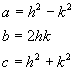Since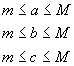we have

•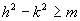, that is the points with coordinates h and k must be 'under' the equilateral hyperbola in its canonical coordinate system with semi-axis √m;
•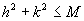, that is the points with coordinates h and k must be internal to the circumference with center at the origin with radius equal to √M;
•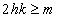, that is the points with coordinates h and k must 'stand over' the equilateral hyperbola with equation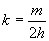;

If, for example, m=25 and M=100, the primitive Pythagorean triples can be obtained by this graph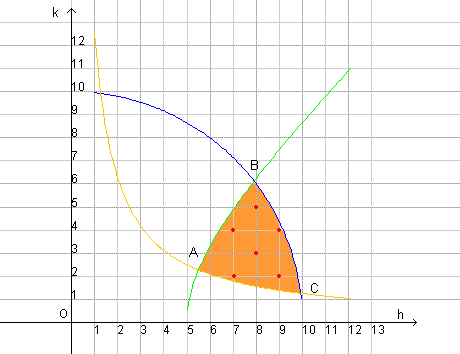by considering the pairs (h,k) corresponding to the red highlighted points in the curvilinear triangle ABC.

• From the pair (7,2) we get a=49-4=45; b=2*7*2=28; c=49+4=53.
• From the pair (7,4) we get a=49-16=33; b=2*7*4=56; c=49+16=65.
• From the pair (8,3) we get a=64-9=55; b=2*8*3=48; c=64+9=73.
• From the pair (8,5) we get a=64-25=39; b=2*8*5=80; c=64+25=89.
• From the pair (9,2) we get a=81-4=77; b=2*9*2=36; c=81+4=85.
• From the pair (9,4) we get a=81-16=64; b=2*9*4=71; c=81+16=97.

last revision: May 2018